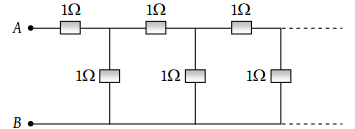# NEET Physics Current Electricity Questions Solved

The equivalent resistance between points A and B of an infinite network of resistances each of 1 Ω connected as shown, is(1) Infinite

(2) 2 Ω

(3) $\frac{1+\sqrt{5}}{2}\Omega$

(4) Zero